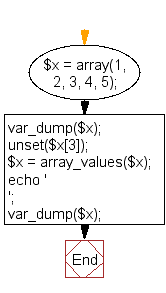﻿ PHP Array Exercise: Delete an element from an array - w3resource# PHP Array Exercises : Delete an element from an array

## PHP Array: Exercise-4 with Solution

\$x = array(1, 2, 3, 4, 5);
Delete an element from the above array. After deleting the element, integer keys must be normalized.

Sample Solution:

PHP Code:

``````<?php
\$x = array(1, 2, 3, 4, 5);
var_dump(\$x);
unset(\$x);
\$x = array_values(\$x);
echo '
';
var_dump(\$x);
?>
```
```

Sample Output:

```array(5) {
=>
int(1)
=>
int(2)
=>
int(3)
=>
int(4)
=>
int(5)
}
array(4) {
=>
int(1)
=>
int(2)
=>
int(3)
=>
int(5)
}
```

Flowchart:PHP Code Editor:

What is the difficulty level of this exercise?

﻿

## PHP: Tips of the Day

PHP: Extract numbers from a string

```\$str = 'In My Cart : 11 12 items';
preg_match_all('!\d+!', \$str, \$matches);
print_r(\$matches);
```